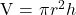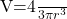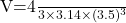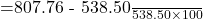## Tennis balls are stored in a cylindrical container with height 21 centimeters and diameter 7 centimeters. Three tennis balls fit in one cont

Question

Tennis balls are stored in a cylindrical container with height 21 centimeters and diameter 7 centimeters. Three tennis balls fit in one container.
a. Find the volume of the container.
b. Find the volume of a tennis ball.
c. Find the percentage of the container taken up by the tennis balls.

in progress 0
3 months 2021-08-18T06:29:53+00:00 1 Answers 0 views 0

1. Solution :

Given that :

Height of the cylinder, h = 21 cm

Diameter of the cylinder, d = 7 cm

Number of balls fits in the cylindrical container = 3

a). The volume of the container is given by= 3.14 x 3.5 x 3.5 x 21

= 807.76 cm cube

b). Volume of each tennis ball is given by :=  179.50 cm cube

c). Volume of three tennis balls = 3 x 179.50

= 538.50 cm cube

Therefore, percentage of the container taken up by the three tennis ball is := 50 %